COMMON MISTAKES
COMMON MISTAKES HOME

ERROR CATEGORIES
ROOTS & EXPONENTS
ALGEBRA
TRIGONOMETRY
VECTORS & MATRICES

MAIN
HOME
TESTS
TUTORIALS
SAMPLE PROBLEMS
COMMON MISTAKES
STUDY TIPS
GLOSSARY
APPLICATIONS
MATH HUMOUR

### COMMON MISTAKES

This section lists common mistakes that students can sometimes make. For ease of reference the errors are divided into four main categories. The Root and Exponent Errors section deals with errors involving exponents and roots. The Algebraic Manipulation Errors section includes errors which may occur when dealing with manipulating expressions. The Trigonometric Errors section deals with common errors involving trigonometry. Finally, the Vectors and Matrices Errors section deal with common errors in vector and matrix operations.

Note that these are listed by ERROR so the formula that appears above the explanation is INCORRECT. The correct formula (if one exists) appears at the end of the explanation.

# Root and Exponent ErrorsLet us assume that this is true. Let a=1 and b=4. Then we have:Since the square root of 5 is not equal to three we can see that you cannot split the root in such a way.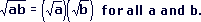Let us assume that the equation is true. Let a=-1 and b=-4. Then we have:This example made use of complex numbers. It can be shown that with the use of complex numbers either a or b can be negative but not both.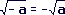Let us assume that the equation is true. Let a=-4. Then we have: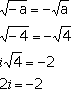This example made use of complex numbers. So keep in mind the negative sign cannot be removed from the square root.

(cx)n = cxn where c is constant.

Let us assume that the equation is true. Let c=2, x=3, and n=2. Then we have:

(cx)n = cxn
((2)(3))2 = 2(32)
62 = 2(9)
36=18

It is quite clear that 36 is not equal to 18 so the assumption that this is true is wrong. The correct formula for removing brackets in this case is (cx)n = cnxn.Let us assume that the equation is true. Let c=8, x=8, and n=3. Then we have: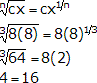As 4 is not equal to 16 this formula is flawed.  The correct formula is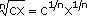.

(x+y)2 = x2+y2

Let us assume that the equation is true. Let x=2 and y=4. Then we have:

(x+y)2 = x2+y2
(2+4)2 = 22+42
62 = 4+16
36 = 20

Since 20 is not equal to 36 (36 being the correct answer) this formula is wrong. The correct formula is (x+y)2 = x2+2xy+y2 because (x+y)2 means (x+y)(x+y).

(-x)2 = -x2

Let us assume that the equation is true. Let x=2. Then we have:

(-x)2 = -x2
(-2)2 = -22
4 = -(22)
4 = -4

This example shows that the negative sign cannot be factored from the brackets when raised to the power 2. The correct way to solve this is (-x)2 = x2.  Note that this property holds for any even power but if the exponent is odd the negative sign can be factored as in the example.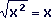Let us assume that the equation is true. Let x = -1. Then we have: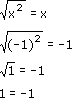The correct way to simplify this is: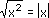# Algebraic Manipulation Errors

x-(a-b) = x-a-b

Let us assume that this is true. Let x=2, a=3, and b=4. Then we have:

x-(a-b) = x-a-b
2-(3-4) = 2-3-4
2-(-1)= -5
2+1 = -5
3 = -5

As can be seen -5 is not equal to 3 so the formula is incorrect.  The error was that the negative sign must be multiplied into both terms in the bracket leaving x-(a-b) = x-a+b.

(ax2+b) / x = ax+b

Let us assume that this is true. Let x=2, a=3, and b=4. Then we have:

(ax2+b) / x = ax+b
(3(22)+4) / 2 = 3(2)+4
(3(4)+4) / 2 = 6+4
16 / 2 = 10
8 = 10

Since 10 does not equal 8 this formula is incorrect.  The error was believing that in dividing by x you only needed to remove x from one term in the numerator. In order to do this EVERY term in the numerator must have an x in it.

ax2 = x solved by dividing both sides by x.  I.E. solve ax = 1

Let us assume that this is true. Let  a=2. Then we have:

ax2 = x
ax = 1
2x = 1
x = 1/2

This seems plausible since we get a value for x and when substitute into the equation it works. However, if we solve it the right way (by subtracting x from both sides so the equation is equal to zero) we will see why dividing by x is not the way to solve the equation.

ax2 = x
ax2-x = 0
x(ax-1) = 0
x(2x-1) = 0

so x = 0 or 2x-1 = 0

x = 0 or 2x-1 = 0
x = 0 or 2x = 1
x = 0 or x = 1/2

Therefore this method gives x = 1/2 as an answer as well but it also gives x = 0 as another possible value for x. This means that the first method is incorrect and the correct way to solve the equation is to subtract x from both sides.

1 / (x+y) = 1/x + 1/y

Let us assume that this is true. Let x=2 and y=3. Then we have:

1 / (x+y) = 1/x + 1/y
1 / (2+3) = 1/2 + 1/3
1/5 = 3/6 + 2/6
1/5 = 5/6

Which is obviously false. It is often better to leave terms of this type in their original form as splitting them up is usually more difficult than evaluating directly.

# Trigonometric Errors

sin(x) = sin(x) (note that the symbol beside the second x is degree not power 0)

In calculus and algebra sin(x) means the sine of x radians not x degrees.  To show an example that these are different, let x=2.  Then we have:

sin(x) = sin(2)0.03489950
sin(x) = sin(2)0.90929743

Even though the value of 2 was used in both equations, the difference between 2 degrees and 2 radians made the answers different. Remember that in calculus and algebra the value will almost always be in RADIANS. But read each problem carefully to be sure you don't make the mistake of assuming the wrong value.

This applies to all trigonometric terms, not just sin(x).

sin(x+y) = sin(x) + sin(y)

Let us assume that this is true. Let x=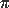and y=/6. Then we have:

sin(x+y) = sin(x) + sin(y)
sin(+/6) = sin() + sin(/6)
sin(7/6) = 0+1/2
-1/2 = 1/2

This shows that the assumed formula is incorrect (since 1/2 is not equal to -1/2).  The correct formula in this situation is
sin(x+y) = sin(x)cos(y)+cos(x)sin(y). Formula for the other trigonometric functions can be found in the Trigonometric Formulas section.

Note that this applies to any trigonometric function and also for '-' instead of '+'.

cos-1(x) = 1 / cos(x)

Let us assume that this is true. Let x=0. Then we have:

cos-1(x) = 1 / cos(x)
cos-1(0) = 1 / cos(0)/2 = 1 / 1/2 = 1

Since/2 is not equal to 1 so the assumption is wrong. This is a common mistake since people often think that because cos2(x) = (cos(x))2 then cos-1(x) should equal (cos(x))-1. However, cos-1(x) denotes the inverse cosine function, also called arccosine, not the cosine function raised to the power -1. See the Inverse Trigonometric Functions section for more details.

Note that this observation applies for any trigonometric function.

# Vector and Matrix Errors

u • v = [u1v1, u2v2, ...]

The dot product gives us a scalar, not another vector. The products are added together, not put into vector components.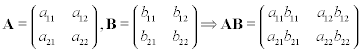Matrix multiplication involves taking dot products of rows from A with columns from B to get the respective row,column entry in AB

1/A, where A is a matrix

There is no such thing as dividing a scalar by a matrix. Division by a matrix does not exist, and what you're probably thinking of/looking for is the inverse of the matrix, A-1.

|Top of Page |

FACULTY HOMEPAGES
Alex Karassev

NIPISSING LINKS
Nipissing University
Nipissing Email
Web Advisor

E-MAIL
Murat Tuncali
Alex Karassev
Vesko Valov
Andrew Dean

QUESTIONS/COMMENTS
 Please forward any questions, comments, or problems you have experienced with this website to Murat Tuncali.